# CSC 270 Survey of Programming Languages C Lecture

• Slides: 28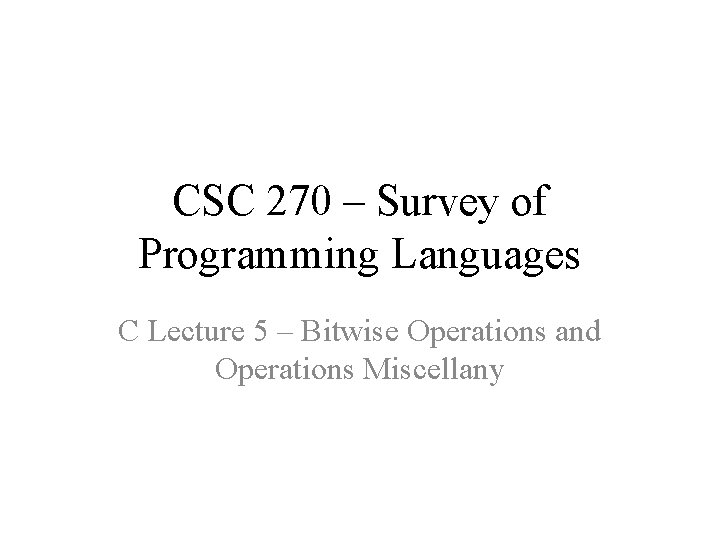CSC 270 – Survey of Programming Languages C Lecture 5 – Bitwise Operations and Operations MiscellanyLogical vs. Bitwise Operations • Logical operations assume that the entire variable represents either true or false. – Combining two integer values using a logical operator produces one result, with the variable representing true or false. • Bitwise operations assume that each bit in the variable’s value represents a separate true or false. – Combining two integer values using a bitwise operators produces 8 (or 16 or 32 or 64) separate bits each representing a true or a false.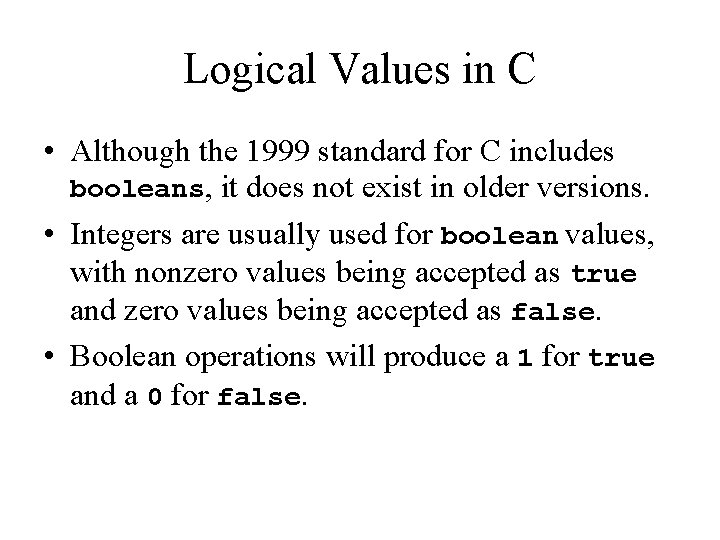Logical Values in C • Although the 1999 standard for C includes booleans, it does not exist in older versions. • Integers are usually used for boolean values, with nonzero values being accepted as true and zero values being accepted as false. • Boolean operations will produce a 1 for true and a 0 for false.&& - Logical AND • The logical AND operator is && and produces a 1 if both operands are nonzero; otherwise, it produces a 0. x y x &&y 0 0 1 1 1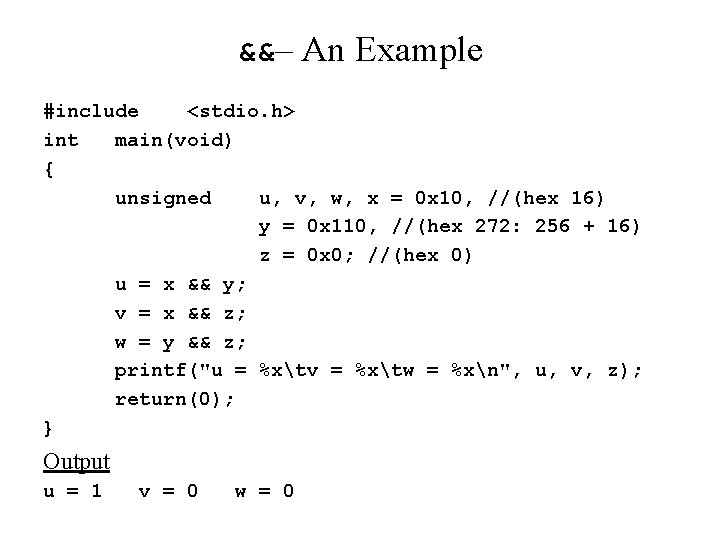&&– An Example #include <stdio. h> int main(void) { unsigned u, v, w, x = 0 x 10, //(hex 16) y = 0 x 110, //(hex 272: 256 + 16) z = 0 x 0; //(hex 0) u = x && y; v = x && z; w = y && z; printf("u = %xtv = %xtw = %xn", u, v, z); return(0); } Output u = 1 v = 0 w = 0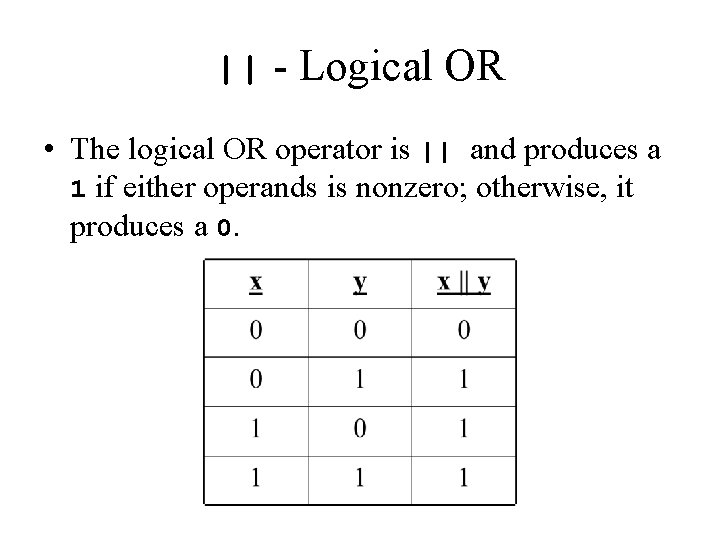|| - Logical OR • The logical OR operator is || and produces a 1 if either operands is nonzero; otherwise, it produces a 0.Logical ||– An Example #include <stdio. h> int main(void) { unsigned u, v, w, x = 0 x 10, y = 0 x 110, z = 0 x 0; u = x || y; v = x || z; w = y || z; printf("u = %xtv = %xtw = %xn", u, v, z); return(0); } Output u = 1 v = 1 w = 1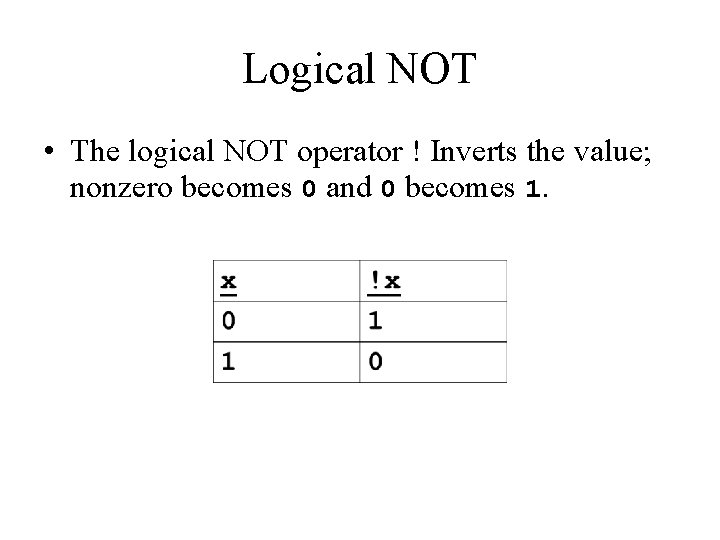Logical NOT • The logical NOT operator ! Inverts the value; nonzero becomes 0 and 0 becomes 1.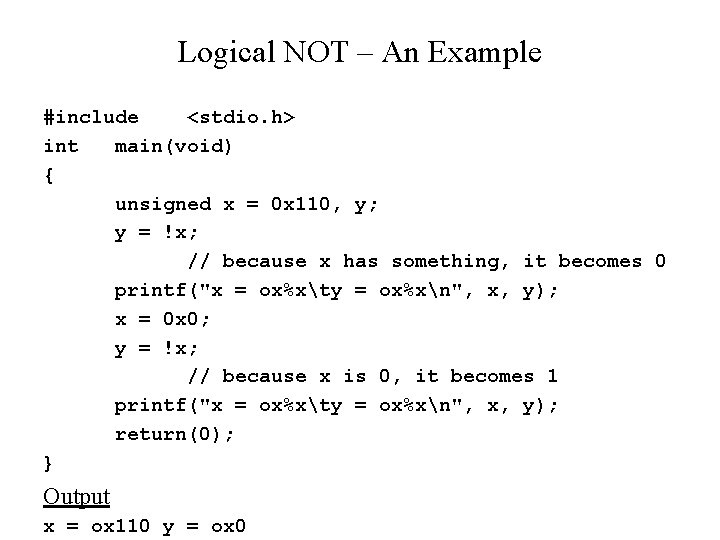Logical NOT – An Example #include <stdio. h> int main(void) { unsigned x = 0 x 110, y; y = !x; // because x has something, it becomes 0 printf("x = ox%xty = ox%xn", x, y); x = 0 x 0; y = !x; // because x is 0, it becomes 1 printf("x = ox%xty = ox%xn", x, y); return(0); } Output x = ox 110 y = ox 0Bitwise Operations • Bitwise operations treat the operands as 8 -bit (or 16 - or 32 -bit) operands. Performing a bitwise AND operation on two 8 -bit integers means that 8 ANDs are performed on corresponding bits. • Example: 00111011 00001111 00001011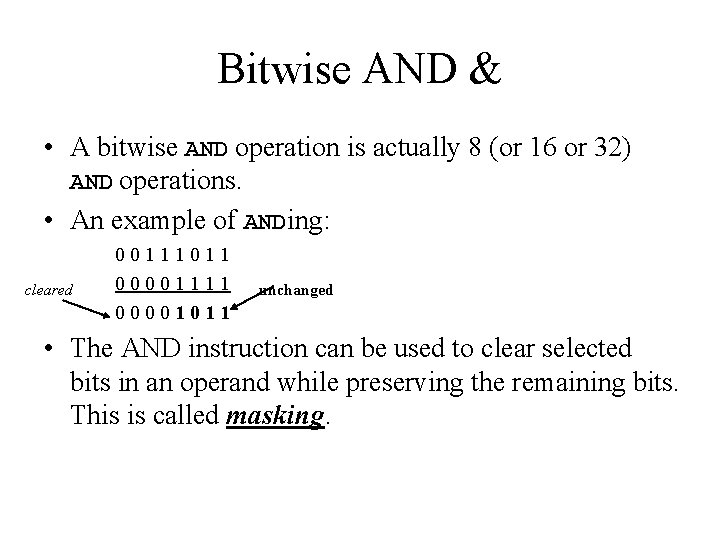Bitwise AND & • A bitwise AND operation is actually 8 (or 16 or 32) AND operations. • An example of ANDing: cleared 00111011 00001111 00001011 unchanged • The AND instruction can be used to clear selected bits in an operand while preserving the remaining bits. This is called masking.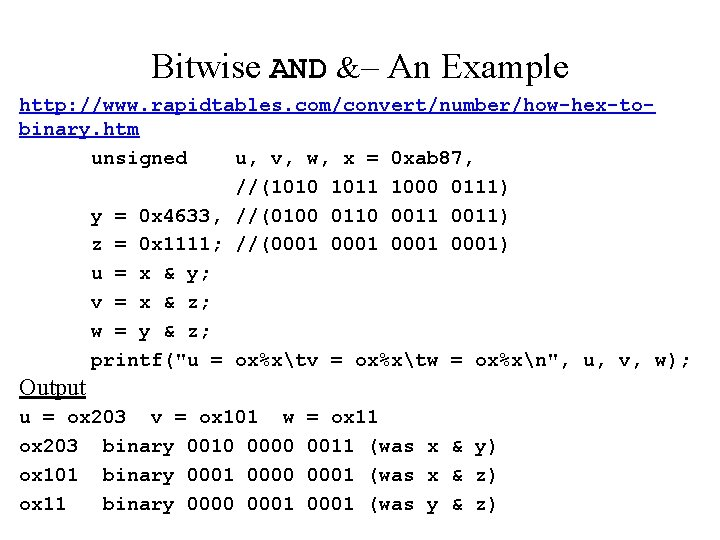Bitwise AND &– An Example http: //www. rapidtables. com/convert/number/how-hex-tobinary. htm unsigned u, v, w, x = 0 xab 87, //(1010 1011 1000 0111) y = 0 x 4633, //(0100 0110 0011) z = 0 x 1111; //(0001 0001) u = x & y; v = x & z; w = y & z; printf("u = ox%xtv = ox%xtw = ox%xn", u, v, w); Output u = ox 203 v = ox 101 w ox 203 binary 0010 0000 ox 101 binary 0001 0000 ox 11 binary 0000 0001 = ox 11 0011 (was x & y) 0001 (was x & z) 0001 (was y & z)Bitwise OR | • A bitwise OR operation is actually 8 (or 16 or 32) OR operations. • An example of ORing: unchanged 00111011 0000111111 set • The OR instruction can be used to set selected bits in an operand while preserving the remaining bits.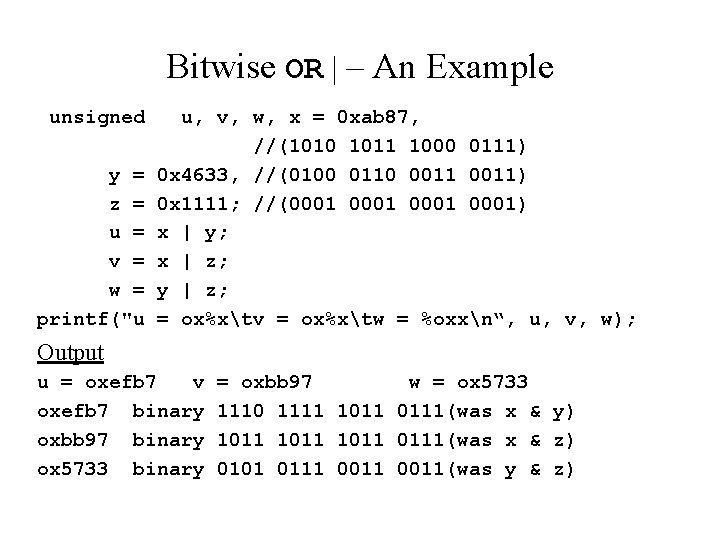Bitwise OR | – An Example unsigned y = z = u = v = w = printf("u u, v, w, x = 0 xab 87, //(1010 1011 1000 0111) 0 x 4633, //(0100 0110 0011) 0 x 1111; //(0001 0001) x | y; x | z; y | z; = ox%xtv = ox%xtw = %oxxn“, u, v, w); Output u = oxefb 7 v oxefb 7 binary oxbb 97 binary ox 5733 binary = oxbb 97 w = ox 5733 1110 1111 1011 0111(was x & y) 1011 0111(was x & z) 0101 0111 0011(was y & z)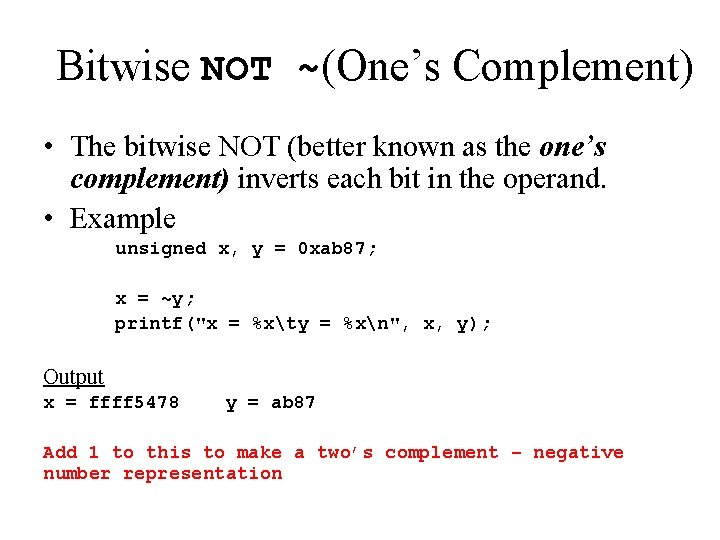Bitwise NOT ~(One’s Complement) • The bitwise NOT (better known as the one’s complement) inverts each bit in the operand. • Example unsigned x, y = 0 xab 87; x = ~y; printf("x = %xty = %xn", x, y); Output x = ffff 5478 y = ab 87 Add 1 to this to make a two’s complement – negative number representation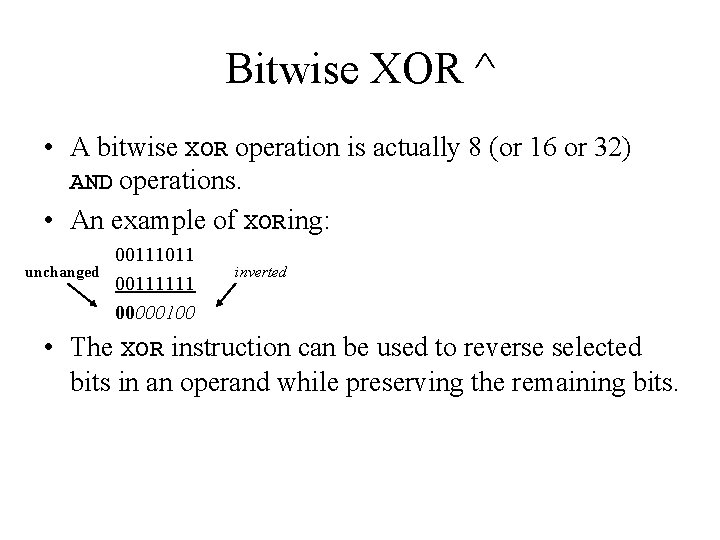Bitwise XOR ^ • A bitwise XOR operation is actually 8 (or 16 or 32) AND operations. • An example of XORing: unchanged 00111011 00111111 00000100 inverted • The XOR instruction can be used to reverse selected bits in an operand while preserving the remaining bits.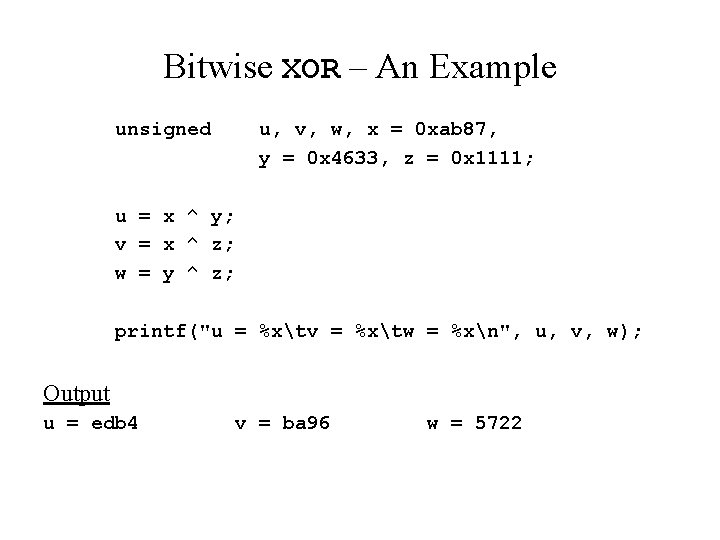Bitwise XOR – An Example unsigned u, v, w, x = 0 xab 87, y = 0 x 4633, z = 0 x 1111; u = x ^ y; v = x ^ z; w = y ^ z; printf("u = %xtv = %xtw = %xn", u, v, w); Output u = edb 4 v = ba 96 w = 5722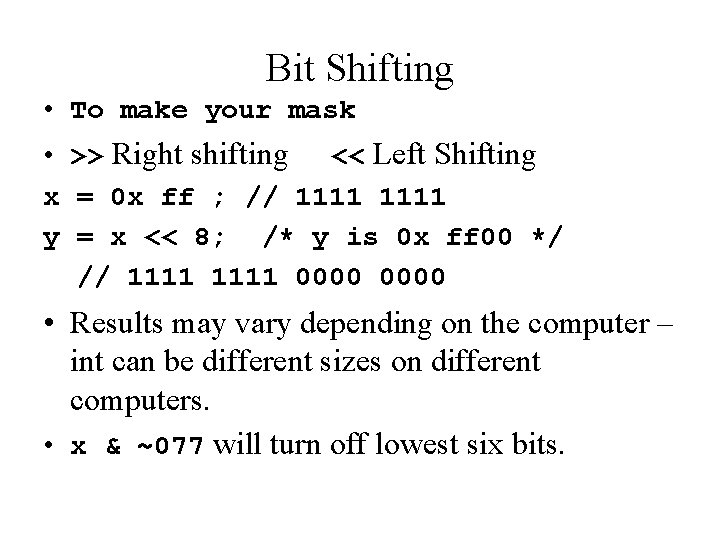Bit Shifting • To make your mask • >> Right shifting << Left Shifting x = 0 x ff ; // 1111 y = x << 8; /* y is 0 x ff 00 */ // 1111 0000 • Results may vary depending on the computer – int can be different sizes on different computers. • x & ~077 will turn off lowest six bits.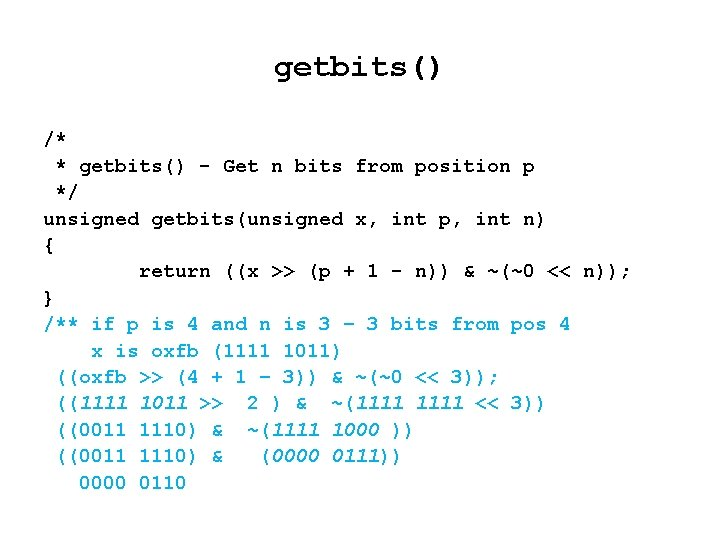getbits() /* * getbits() - Get n bits from position p */ unsigned getbits(unsigned x, int p, int n) { return ((x >> (p + 1 - n)) & ~(~0 << n)); } /** if p is 4 and n is 3 – 3 bits from pos 4 x is oxfb (1111 1011) ((oxfb >> (4 + 1 – 3)) & ~(~0 << 3)); ((1111 1011 >> 2 ) & ~(1111 << 3)) ((0011 1110) & ~(1111 1000 )) ((0011 1110) & (0000 0111)) 0000 0110Assignment Operators • An assignment operator is just another operator in C. • We can rewrite i = i + 2; as or i = i + x * y; as i += 2; i += x * y; • Similarly, there are -=, *=, /=, etc.Assignment Operators • Caution! i *= 2 + y; is rewritten as i = i * (2+y); NOT i = (i *2) + y;bitcount() /* * bitcount() - Count 1 s in x */ int bitcount(unsigned x) { int b; for (b = 0; x != 0; x >>= 1) if (x & 01) b++; return(b); }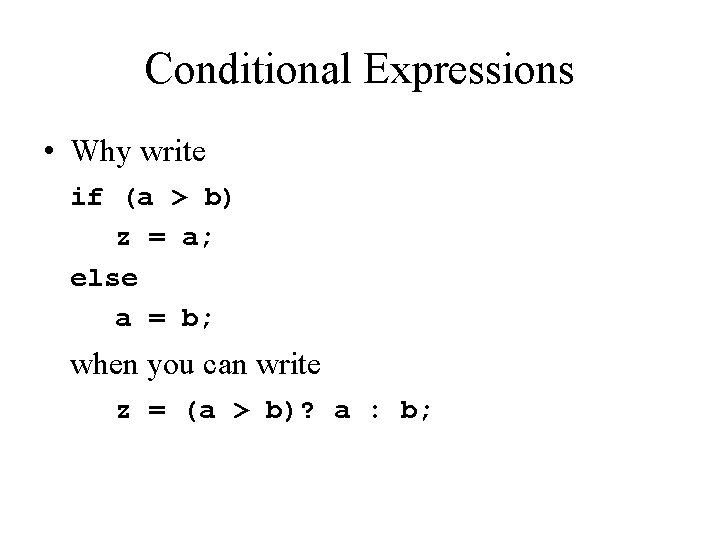Conditional Expressions • Why write if (a > b) z = a; else a = b; when you can write z = (a > b)? a : b;Conditional Expressions • The general form is expression 1? expression 2: expression 3; when expression 1 is nonzero, expression 2 is evaluated. Otherwise expression 3 is evaluated. • The usual rules of conversion are in effect. int i, j, a, b; float x; … … i = (a > b)? j: x;Conditional Expressions • If this useful? YES!! z = (a > b)? a : b; x = (x > 0)? x : -x; /* z = max (a, b); */ /* x = abs(x) */ /* Print 5 values to a line */ for (i = 0; i < MAXSIZE; i++) printf("%d%c", x[i], i % 5 == 4? 'n': 't');Operator Precedence Operator () [] ->. Associativity ! ~ ++ -- -unary (type) * (ptr) & (address) sizeof right to left * / left to right + - left to right << >> left to right < == left to right % <= > >= != left to right & (bitwise AND) left to right ^ (bitwise XOR) left to right | (bitwise OR) left to rightBitwise Summary • • Bitwise operators act on bits inside the number 1 hex digit = 4 binary digits Mask – the number set for comparison & - used to clear bits – 1 = both true; – 0 in the mask clears and 1 preserves original • | - used to set bits – 1= either true; – 1 in the mask sets to 1 and 0 preserves original • ^ - used to reverse bits – 1 = only one true; – 1 in the mask reverses bits and 0 preservesBitwise Summary • ~ one’s complement – reverses every bit • Set your mask using shifting § << a number : left shift by the number and zero fill § >> a number : right shift by the number and zero fill § Extra gift – ternary operator – embedded if/else: § (a > b)? j: x; If a is greater than b then replace expression with j, otherwise replace with x.